# 1.4 The Model of Computation

In this book, we will analyze the theoretical running times of operations on the data structures we study. To do this precisely, we need a mathematical model of computation. For this, we use the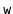-bit word-RAM model. RAM stands for Random Access Machine. In this model, we have access to a random access memory consisting of cells, each of which stores a-bit word. This implies that a memory cell can represent, for example, any integer in the set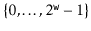.

In the word-RAM model, basic operations on words take constant time. This includes arithmetic operations (,,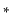,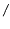,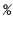), comparisons (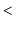,,,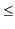,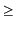), and bitwise boolean operations (bitwise-AND, OR, and exclusive-OR).

Any cell can be read or written in constant time. A computer's memory is managed by a memory management system from which we can allocate or deallocate a block of memory of any size we would like. Allocating a block of memory of size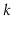takes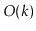time and returns a reference (a pointer) to the newly-allocated memory block. This reference is small enough to be represented by a single word.

The word-sizeis a very important parameter of this model. The only assumption we will make aboutis the lower-bound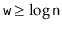, where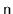is the number of elements stored in any of our data structures. This is a fairly modest assumption, since otherwise a word is not even big enough to count the number of elements stored in the data structure.

Space is measured in words, so that when we talk about the amount of space used by a data structure, we are referring to the number of words of memory used by the structure. All of our data structures store values of a generic type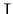, and we assume an element of typeoccupies one word of memory. (In reality, we are storing references to objects of type, and these references occupy only one word of memory.)

The-bit word-RAM model is a fairly close match for the (32-bit) Java Virtual Machine (JVM) when. The data structures presented in this book don't use any special tricks that are not implementable on the JVM and most other architectures.

opendatastructures.org